Specimen of the graphics that only require 1 input variable and that, up to now, are being considered by the brinton’s wideplot(), longplot() and plotup() functions.

Logical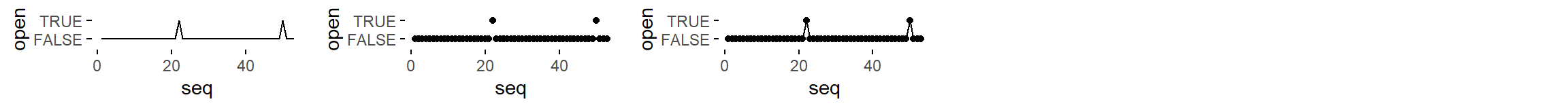lgc = c('line graph', 'point graph', 'point-to-point graph')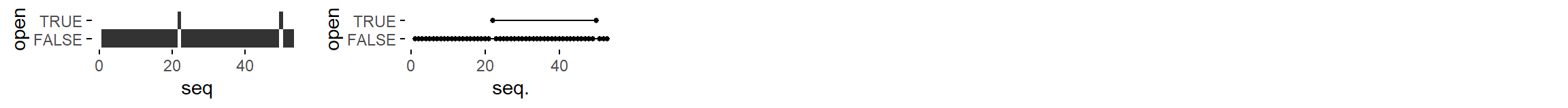lgc = c('tile plot', 'linerange graph')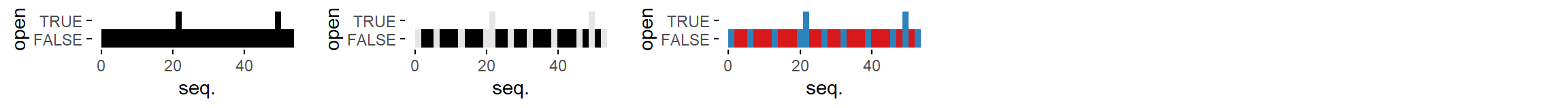lgc = c('binned heatmap', 'bw binned heatmap', 'color binned heatmap')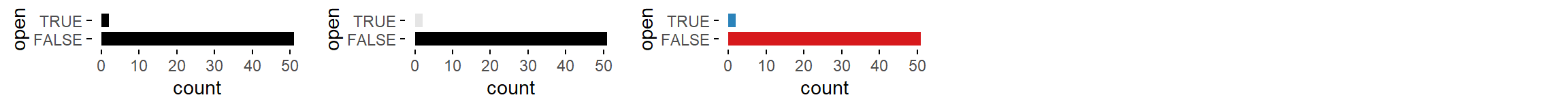lgc = c('bar graph', 'bw bar graph', 'color bar graph')

Ordered factor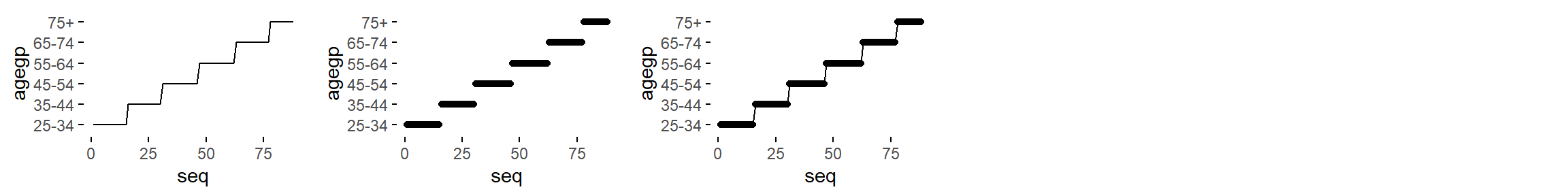ord = c('line graph', 'point graph', 'point-to-point graph')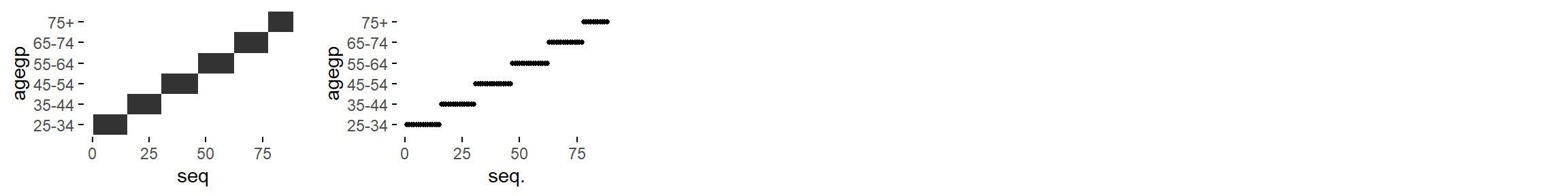ord = c('tile plot', 'linerange graph')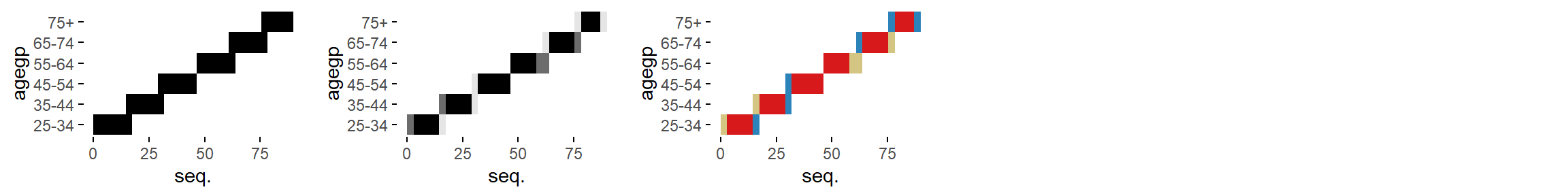lgc = c('binned heatmap', 'bw binned heatmap', 'color binned heatmap')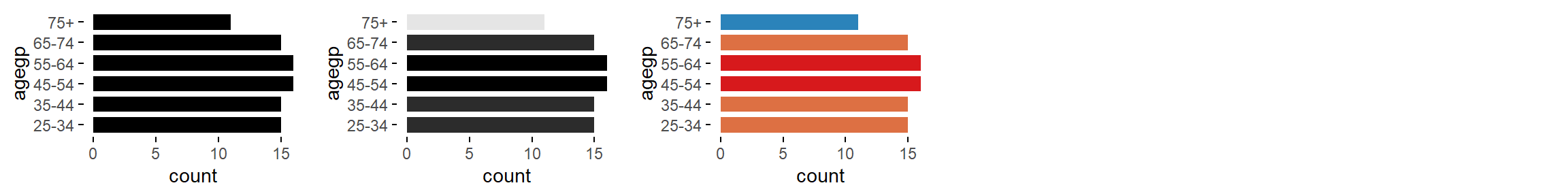ord = c('bar graph', 'bw bar graph', 'color bar graph')

Factor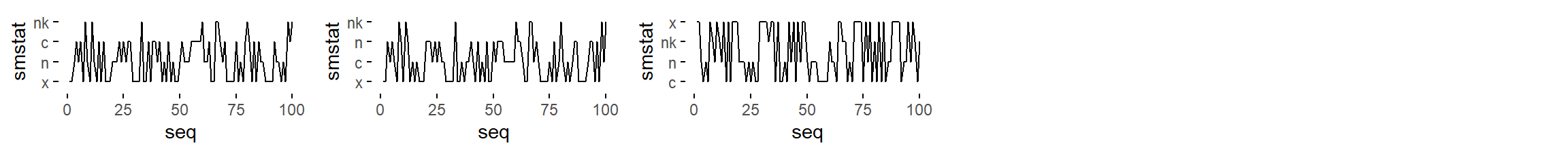fac = c('line graph', 'freq. reordered line graph', 'alphab. reordered line graph')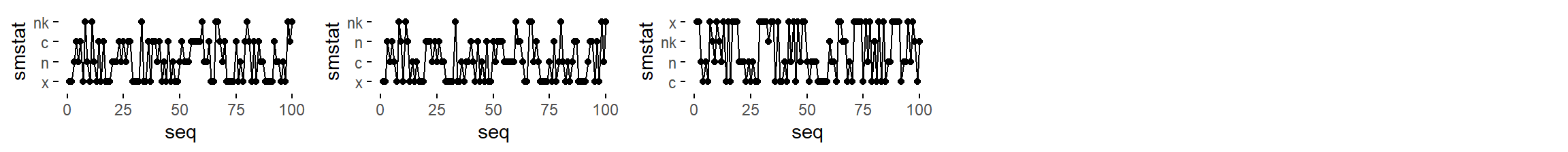fac = c('point-to-point graph', 'freq. reordered point-to-point graph', 'alphab. reordered point-to-point graph')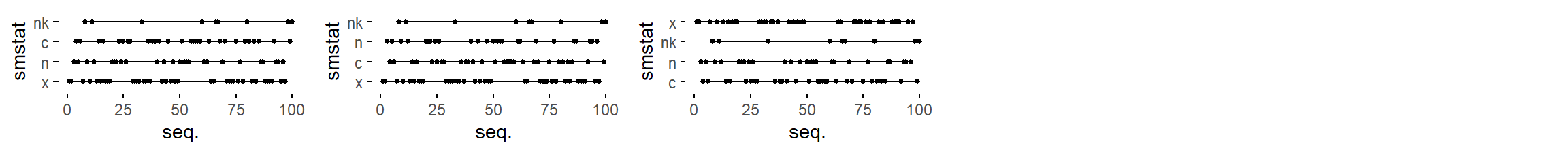fac = c('linerange graph', 'freq. reordered linerange graph', 'alphab. reordered linerange graph')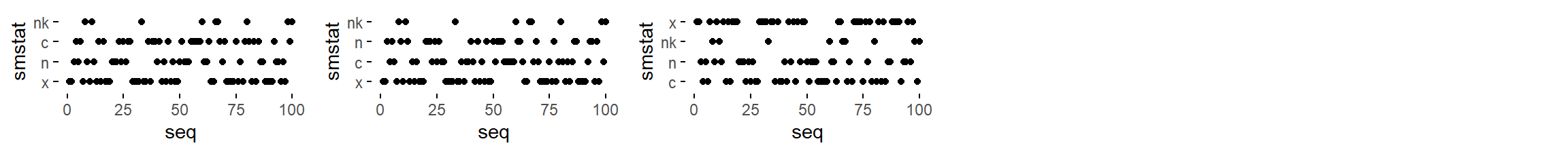fac = c('point graph', 'freq. reordered point graph', 'alphab. reordered point graph')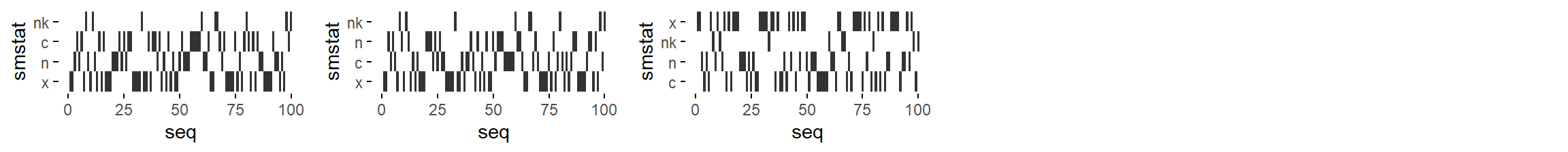fac = c('tile plot', 'freq. reordered tile plot', 'alphab. reordered tile plot')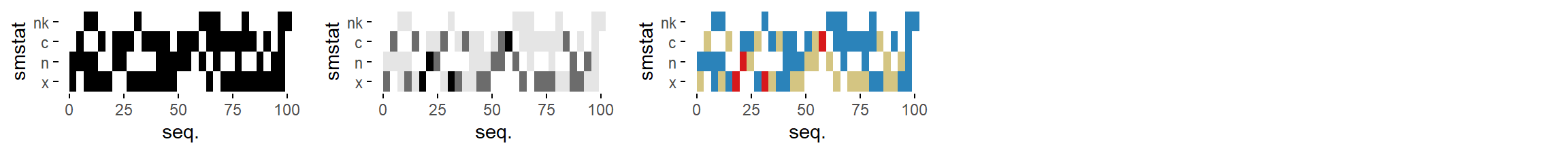fac = c('binned heatmap', 'bw binned heatmap', 'color binned heatmap')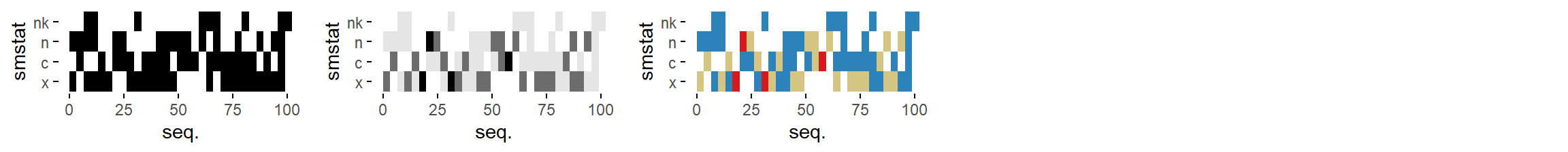fac = c('freq. reordered binned heatmap',
'bw freq. reordered binned heatmap',
'color freq. reordered binned heatmap')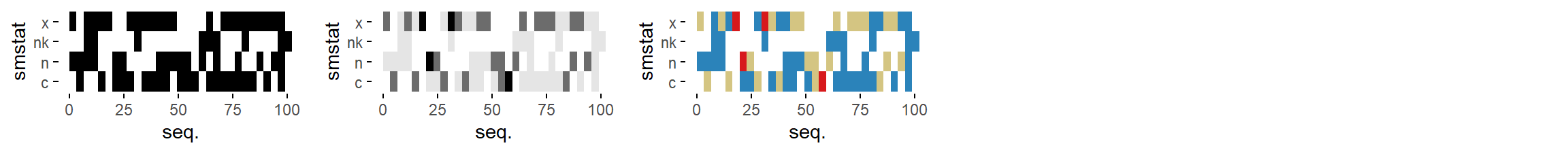fac = c('alphab. reordered binned heatmap',
'bw alphab. reordered binned heatmap',
'color alphab. reordered binned heatmap')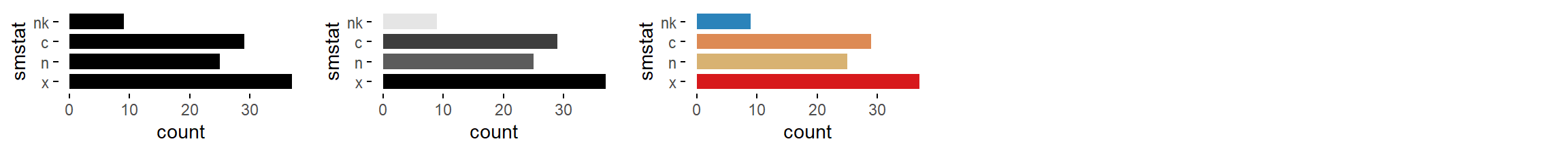fac = c('bar graph', 'bw bar graph', 'color bar graph')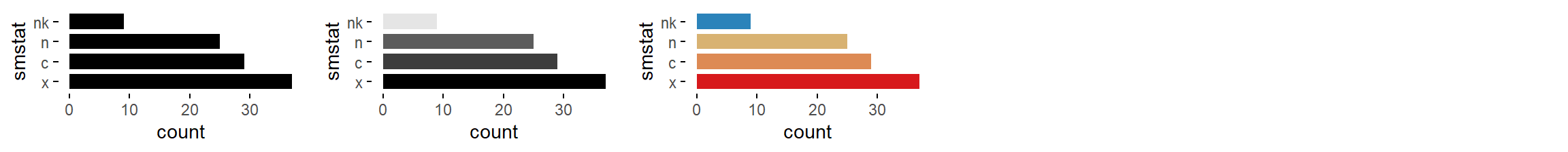fac = c('freq. reordered bar graph', 'bw freq. reordered bar graph', 'color freq. reordered bar graph')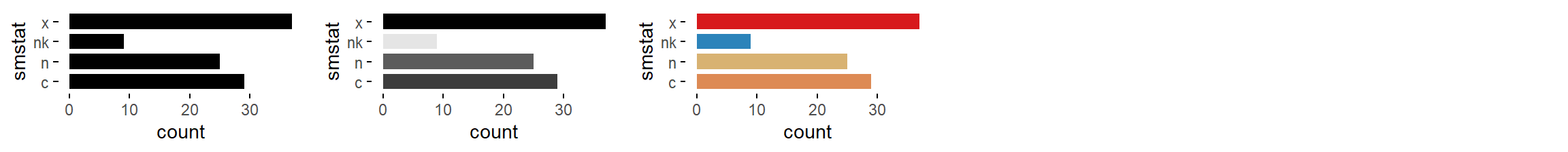fac = c('alphab. reordered bar graph', 'bw alphab. reordered bar graph', 'color alphab. reordered bar graph')

Character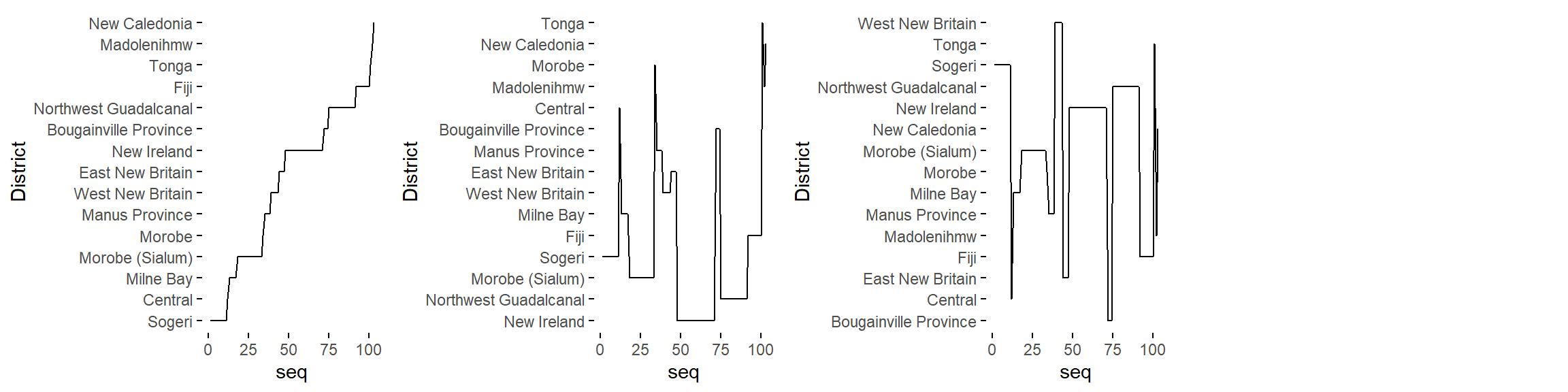cha = c('line graph', 'freq. reordered line graph', 'alphab. reordered line graph')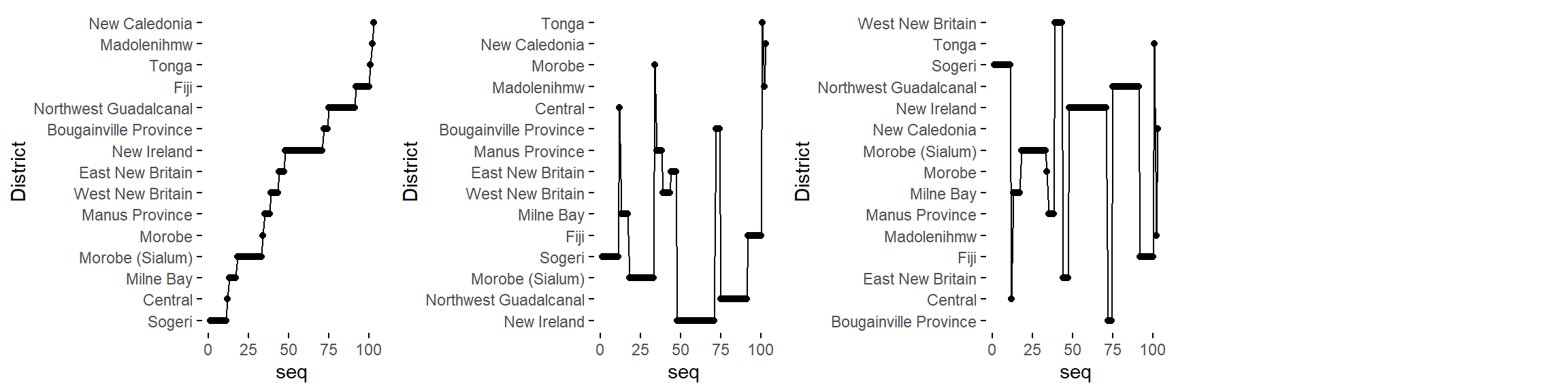cha = c('point-to-point graph', 'freq. reordered point-to-point graph', 'alphab. reordered point-to-point graph')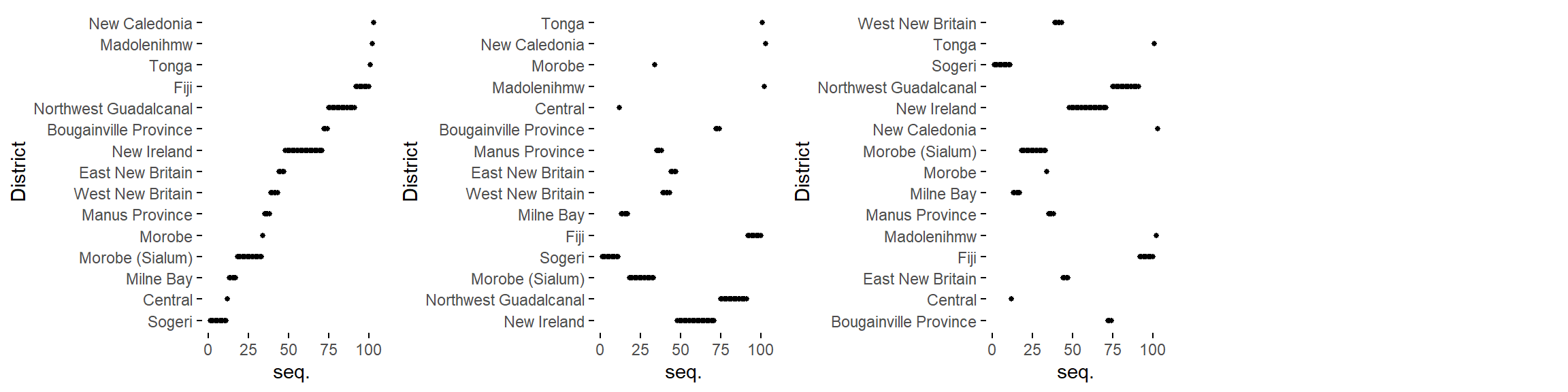cha = c('linerange graph', 'freq. reordered linerange graph', 'alphab. reordered linerange graph')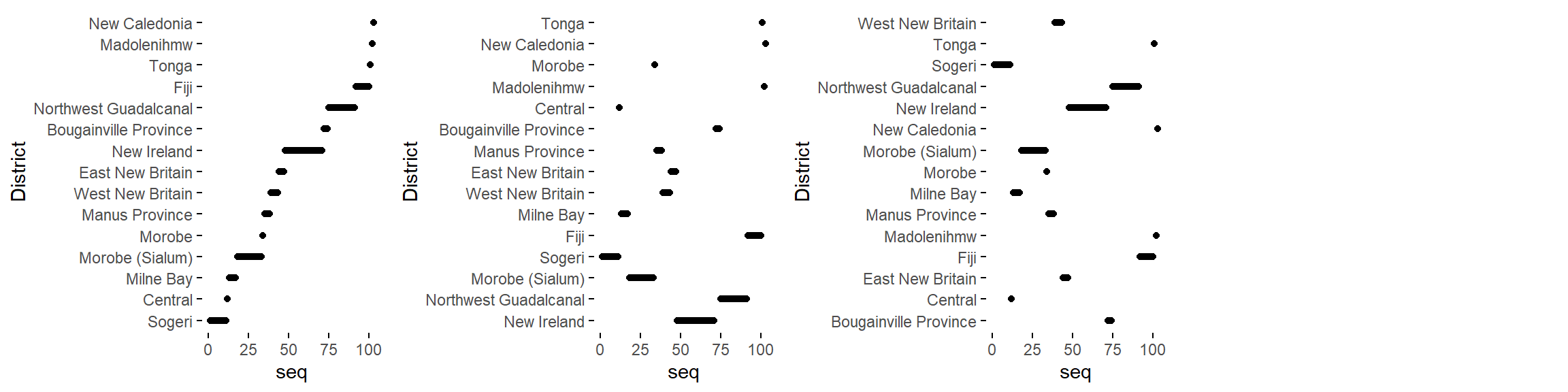cha = c('point graph', 'freq. reordered point graph', 'alphab. reordered point graph')